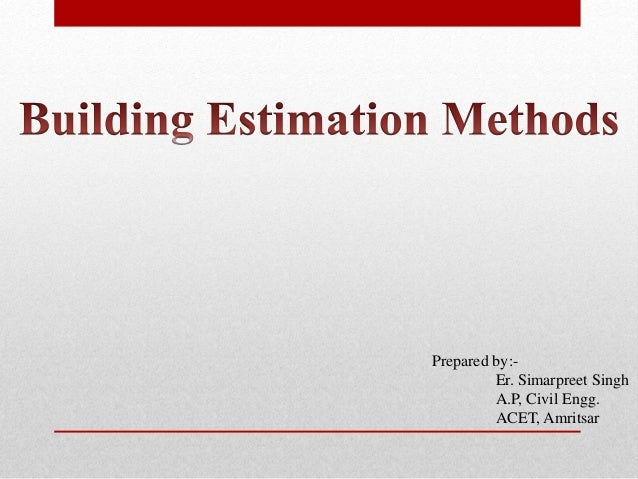Successfully reported this slideshow.Upcoming SlideShare
×

# Building estimation methods

33,965 views

Published on

Brief comparison between Separate Wall Method and Center Line Method of estimation of buildings in Civil Engineering

Published in: Education
• Full Name
Comment goes here.

Are you sure you want to Yes No• very well explained

Are you sure you want to  Yes  No

### Building estimation methods

1. 1. Prepared by:- Er. Simarpreet Singh A.P, Civil Engg. ACET, Amritsar
2. 2. TABLE OF CONTENTS  One Room Building  Concrete work in foundation (Center line and separate wall method)  Brickwork in footing (Center line and separate wall method)  Brickwork in walls (Center line and separate wall method)  Two Rooms Building  Concrete work in foundation (Center line and separate wall method)
3. 3. 5m 4m 0.3m 0.3m 0.5m 0.6m 0.9m 0.4m 0.6m 3.5m Section at A-A’ and B-B’ Thickness of footing and foundation is 30 cm each A A’ B B’ Plan
4. 4. 0.9 m 5.3m 4.3 m Total Center line = 5.3+5.3+4.3+4.3=19.2 m Width = 0.9 m Total Qty of concrete in foundation= (19.2×0.9×0.3) cum Center Line Method
5. 5. 0.9 m 5.3m 6.2m 4.3 m 3.4 m Separate Wall Method Width = 0.9 m Total Qty of concrete in fdn= (2×6.2×0.9×0.3) + (2×3.4×0.9×0.3)cum Long Wall = 5.3+0.9=6.2 m Short Wall = 4.3-0.9=3.4 m
6. 6. 0.6 m 5.3m 4.3 m Total Center line = 5.3+5.3+4.3+4.3=19.2 m Width = 0.6 m Total Qty of brickwork in Ist footing= (19.2×0.6×0.3) cum Center Line Method
7. 7. 0.6 m 5.3m 5.9 m 4.3 m 3.7 m Width = 0.6 m Separate Wall Method Long Wall = 5.3+0.6=5.9 m Short Wall = 4.3-0.6=3.7 m Total Qty of brickwork in Ist footing= (2×5.9×0.6×0.3) + (2×3.7×0.6×0.3)cum
8. 8. 5.3m 4.3 m 0.3 m Total Center line = 5.3+5.3+4.3+4.3=19.2 m Width = 0.3 m Total Qty of brickwork in walls= (19.2×0.3×3.5) cum Center Line Method
9. 9. 5.6 m 5.3m 4.3 m 4 m 0.3 m Width = 0.3 m Separate Wall Method Long Wall = 5.3+0.3=5.6 m Short Wall = 4.3-0.3=4.0 m Total Qty of brickwork in walls= (2×5.6×0.3×3.5) + (2×4.0×0.3×3.5)cum
10. 10. 5m 4m 0.3m 0.3m 0.5m 0.6m 0.9m 0.4m 0.6m 3.5m Section at A-A’ and B-B’ Thickness of footing and foundation is 30 cm each A A’ B B’ Plan
11. 11. Center Line Method Total Center line = 5.3+5.3+5.3+5.3+4.3+4.3+4.3=34.1 m Width = 0.9 m Total Qty of concrete in fdn= (34.1×0.9×0.3) 5.3m 5.3m 4.3 m 0.9 m Intermediate wall – (2×0.9×(0.9/2) ×0.3)cum
12. 12. Separate Wall Method Long Wall = 5.3+5.3+0.9=11.5 m Short Wall = 4.3-0.9=3.4 m Width = 0.9 m Total Qty of concrete in fdn= (2×11.5×0.9×0.3) 5.3m 5.3m 4.3 m 0.9 m 11.5 m 3.4m Intermediate wall + (3×3.4×0.9×0.3)cum